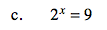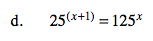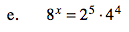### Home > A2C > Chapter 3 > Lesson 3.2.2 > Problem3-110

3-110.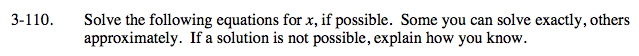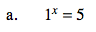11 = 1
13 = 1
12 = 1
14 = 1

No solution. Explain why.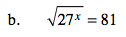Rewrite in exponential form.

81 and 27 can both be rewritten as powers of 3.

Simplify the exponents and solve for x.

$x=\frac{8}{3}=2.\overline{6}$